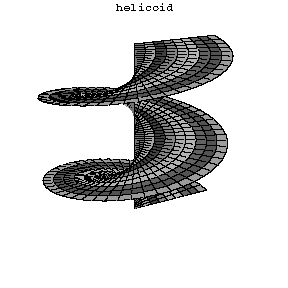# Helicoid

A ruled surface described by a straight line that rotates at a constant angular rate around a fixed axis, intersects the axis at a constant angle $\alpha$, and at the same time becomes gradually displaced at a constant rate $k$ along this axis. The equation of a helicoid in parametric form is $x = \rho \cos t \quad y = \rho \sin t \quad z = \rho \arctan \alpha + kt\, .$ If $\alpha = \pi/2$ the helicoid is called straight or right (cf. Fig. 1), otherwise it is called oblique. However, it is common to use the word "helicoid" without specification for the straight helicoid. Every straight helicoid is a minimal surface, i.e. it has mean curvature zero. In fact the straight helicoid can be characterized by the property of being the only minimal ruled suface. More precisely we have the following
Theorem If $\Sigma\subset \mathbb R^3$ is a connected $2$-dimensional ruled minimal surface, then it is a subset of a straight helicoid or a subset of a plane.